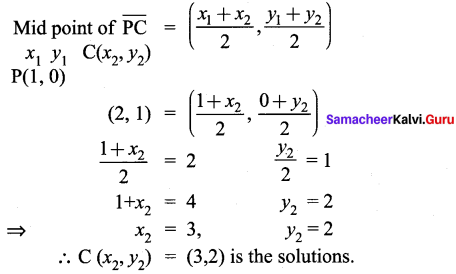# Samacheer Kalvi 9th Maths Solutions Chapter 5 Coordinate Geometry Ex 5.4

## Tamilnadu Samacheer Kalvi 9th Maths Solutions Chapter 5 Coordinate Geometry Ex 5.4

Question 1.
Find the coordinates of the point which divides the line segment joining the points
A (4, -3) and B (9, 7) in the ratio 3 : 2.
Solution: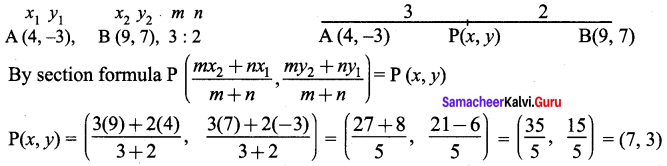Question 2.
In what ratio does the point P (2, -5) divide the line segment joining A (-3, 5) and B (4, -9).
Solution: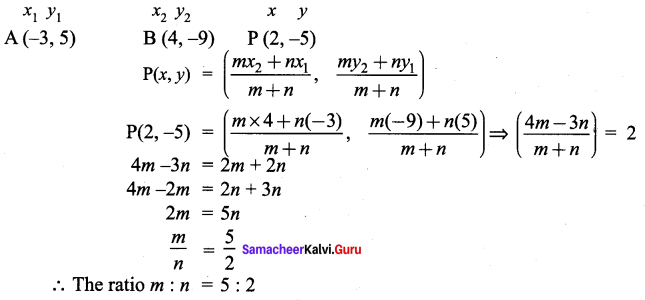Question 3.
Find the coordinates of a point P on the line segment joining A(1, 2) and B (6, 7) in such a way that AP = $$\frac{2}{5}$$AB.
Solution: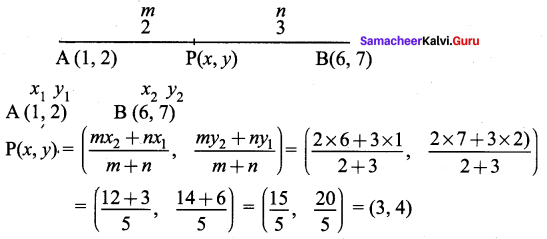Question 4.
Find the coordinates of the points of trisection of the line segment joining the points A (-5, 6) and B (4, -3).
Solution: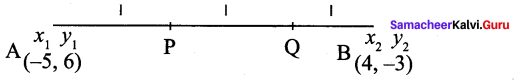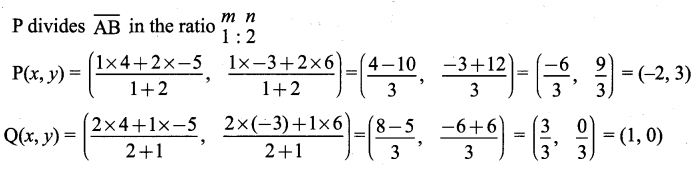Question 5.
The line segment joining A(6, 3) and B(-1, -4) is doubled in length by adding half of AB to each end. Find the coordinates of the new end points.
Solution: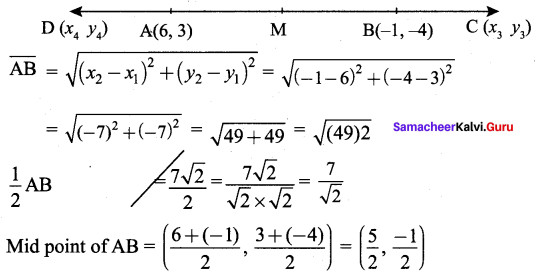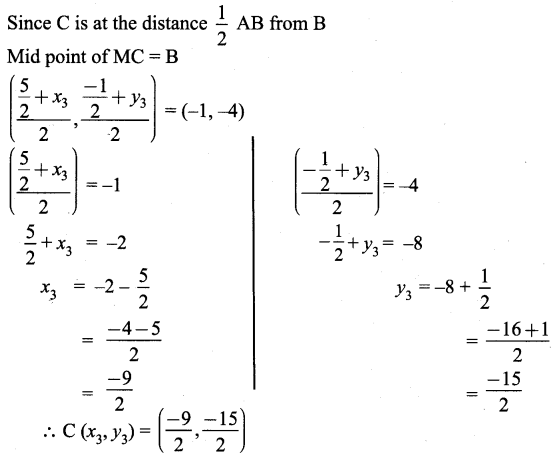Similarly by Mid point of DM = A(6, 3)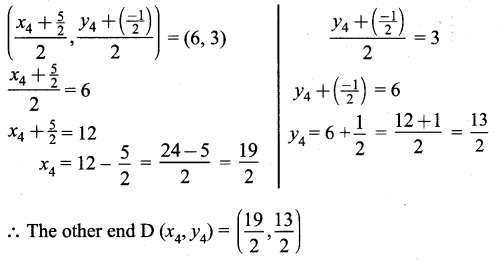Question 6.
Using section formula, show that the points A(7, -5), B(9, -3) and C(13, 1) are collinear.
Solution: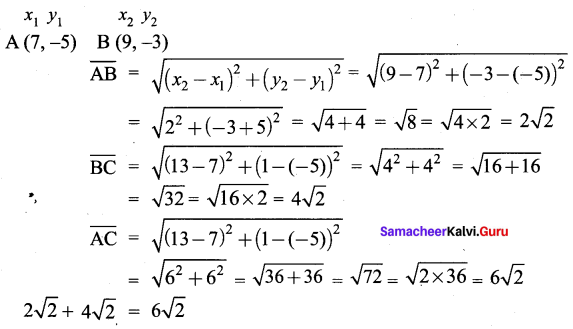∴ AB + BC = AC, Here B is the common Point. ∴ A, B, C are collinearQuestion 7.
A line segment AB is increased along its length by 25% by producing it to C on the side of B. If A and B have the coordinates(-2, -3) and (2, 1) respectively, then find the coordinates of C.
Solution: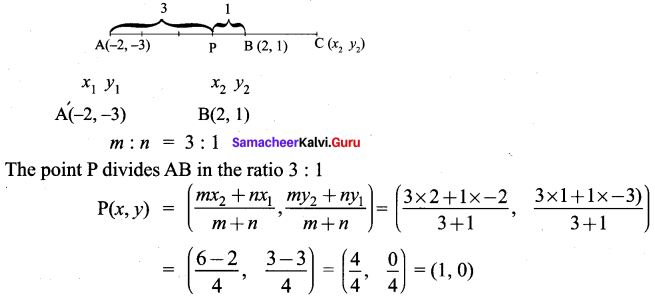P is at 25% distance from B on its left and C is at 25% distance from B on its right.
∴ B is the mid point of PC